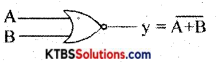# 1st PUC Electronics Previous Year Question Paper March 2014 (South)

Students can Download 1st PUC Electronics Previous Year Question Paper March 2015 (North), Karnataka 1st PUC Electronics Model Question Papers with Answers helps you to revise the complete syllabus.

## Karnataka 1st PUC Electronics Previous Year Question Paper March 2015 (North)

Time: 3.15 Hours
Max Marks: 70

Note :

1. The question paper contains four parts.
2. Part – A is compulsory, Part – D contains two subparts (a) problems (b) essay-type questions.
3. Draw the circuit diagram, wherever necessary.

PART-A

Answer all questions. Each question carries one mark. (10 x 1 = 10)

Question 1.
Expand EPROM.

Question 2.
What is the internal resistance of an ideal voltage source?
Rs = 0Ω.

Question 3.
What is a Sphygmomanometer?
It measures arterial (blood) pressure.

Question 4.
Draw the circuit symbol of LDR.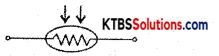Question 5.
What is the Forbidden energy gap?
The separation between the conduction band and valence band is called the Forbidden energy gap.Question 6.
Draw the output waveform of the series positive clipper.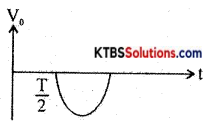Question 7.
Name the Majority charge that carries an NPN transistor.
Electrons.

Question 8.
Write the Binary equivalent of Hexadecimal number (FED)16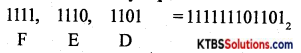Question 9.
Name the Logic gate symbol shown below.
NOT gate.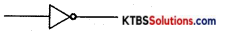Question 10.
What is the important specification of a Resistor?
Power rating.

PART-B

Answer any five questions. (5 × 2 = 10)

Question 11.
Mention any two properties of Electric charge.
Properties of Electric Charge

1. Like charges repel and unlike charges attract.
2. Charges can neither be created nor destroyed.
3. Charges always reside on the outer surface of the conductor.Question 12.
Find the current flowing through 20 resistors using Superposition Theorem.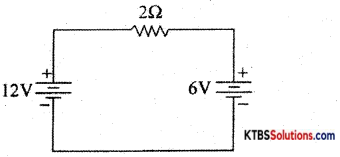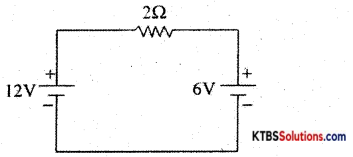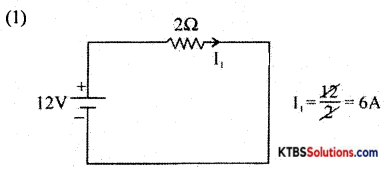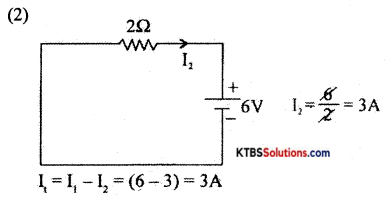Question 13.
Draw the growing current graph in an RL transient circuit indicating the Time Constant.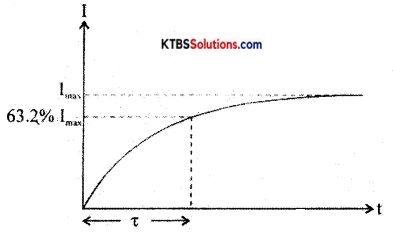Question 14.
Mention any two properties of semiconductors.
Semiconductor properties

1. They are tetravalent.
2. They are perfect insulators at absolute zero-degree temperatures.
3. They have a negative temperature coefficient of resistance.

Question 15.
Draw the circuit symbol of varactor diode and write one application of ¡t.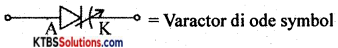Application: FM generator, TV receiver, AFC.Question 16.
Derive an expression for an in terms of
IE = IB + Ic
Dividing both sides by Ic
$$\frac{\mathrm{I}_{\mathrm{E}}}{\mathrm{I}_{\mathrm{C}}}=\frac{\mathrm{I}_{\mathrm{B}}}{\mathrm{I}_{\mathrm{C}}}+1$$
$$\frac{1}{\alpha}=\frac{1}{\beta}+1$$
$$\frac{1}{\alpha}=\frac{1+\beta}{\beta}$$
α = $$\frac{\beta}{1+\beta}$$

Question 17.
Perform subtraction of the binary numbers (1011)2 – (0100)2 using 2’s complement method.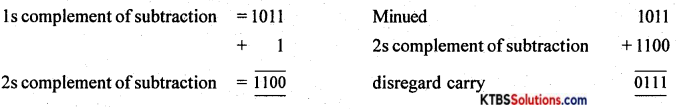Question 18.
For the following Truth-Table, write the logic gate name for Y and write its output expression.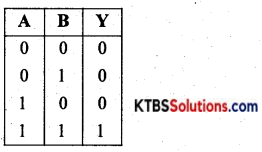And Gate

PART-C

Answer any five questions. Each question carries three marks. (5 × 3 = 15)

Question 19.
Give a brief note on the application of ELECTRONICS in day-to-day life.
Applications:

1. Communication Electronics
2. Industrial Electronics
3. Defense Electronics
4. Medical Electronics

Question 20.
State and Explain Kirchoff’s Current Law.
Statement of KCL
Explanation Σ I incoming = Σ I outgoing.Question 21.
What are the advantages of a Digital Thermometer?
Digital Thermometer

2. Easier to handle
3. Readings can be measured quickly

Question 22.
Derive an expression for total capacitance when two capacitors are connected in parallel.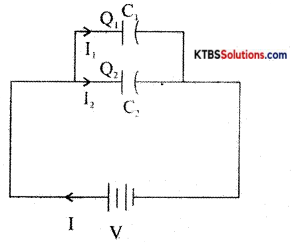QT = Q1 + Q2
CTV = C1V + C2V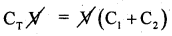CT = C1 + C2

Question 23.
Compare P and N-type of semiconductors.

 P-type N-type (1) By adding trivalent dopants to pure semiconductors, p-type is produced. (1) By adding pentavalent dopants to pure semiconductors, n-type is produced. (2) Acceptors (2) Donors (3) Holes are majority carriers (3) Electrons are the majority carriers.

Question 24.
Explain the working of Bridge Rectifier Shunt Capacitor Filter Circuit.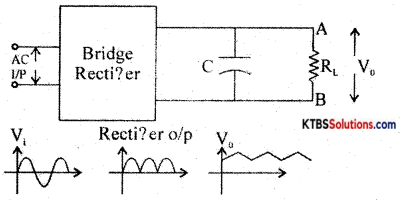Fig. shows the circuit of the Shunt Capacitor Bridge Rectifier with a Wave diagram. A capacitor connected in parallel to the load removes all the a.c (ripple) components and hence only D.C or average value reaches the load.Question 25.
Calculate the Emitter current and α of a transistor having IB = 10mA and IE = 5mA.
IB = 10mA, Ic = 5mA
IE = IB + Ic = (0.01 + 5)mA = 5.01 mA
α = $$\frac{\mathrm{I}_{\mathrm{C}}}{\mathrm{I}_{\mathrm{E}}}=\frac{5 \mathrm{~m} \mathrm{~A}}{5.01 \mathrm{~mA}}$$ = 0.09

Question 26.
Mention any three advantages of using PCB

1. The circuit will be neat without extra wires.
2. Higher density components are placed.
3. The cost of production reduces on a larger volume of circuit boards.
4. Assembled circuits can be easily tested.

PART-D

(a) Answer any three of the following. (3 × 5 = 15)

Question 27.
Convert the circuit into Thevenin’s equivalent circuit and find the current IL through RL.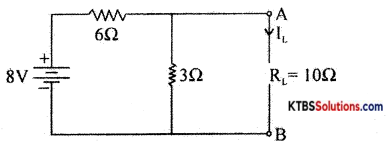VTH = $$\frac{18}{6+3}$$ × 3 = 6 Volts
RTH = 6 ∥ 3 = 2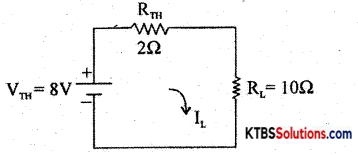VTH = $$\frac{\mathrm{V}_{\mathrm{TH}}}{\mathrm{R}_{\mathrm{TH}}+\mathrm{R}_{\mathrm{L}}}=\frac{6}{(2+10)}$$ = 0.5AQuestion 28.
A transformer having an output power of 4KW and efficiency of 85% has primary voltage= 22kV and secondary voltage = 220V. Is it a step up or step down transformer? Calculate the voltage ratio and the number of turns in primary ¡f the secondary has 28 turns. Calculate the current in the primary.
1. Voltage Ratio = $$\frac{V_{\mathrm{s}}}{\mathrm{V}_{\mathrm{P}}}=\frac{220}{2000}=\frac{1}{100}$$ = 100

2. Step down transformer.

3. Turn ratio = $$\frac{\mathrm{N}_{\mathrm{S}}}{\mathrm{N}_{\mathrm{P}}}=\frac{1}{100}=\frac{28}{\mathrm{~N}_{\mathrm{P}}}=\frac{1}{100}$$
Np = 2800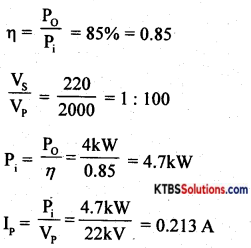Question 29.
A 230V to (15-0-15)V stepdown transformer is used in a center tapper FWR (Full Wave Rectifier) connected to a load of 2000. Determine the following.
(1) Maximum value of the output voltage of the transformer.
(2) D.C. output voltage.
(4) D.C. Output power delivered to the load.
(5) PIV of the diode
V= 15V

1. Vm = $$\sqrt{2}$$ × 15 =21.21V
2. VDc = $$\frac{2 \mathrm{~V}_{\mathrm{m}}}{\pi}$$ = 13.509
3. IDc = $$\frac{\mathrm{V}_{\mathrm{DC}}}{\mathrm{R}_{\mathrm{L}}}$$ = 67.5mA
4. Po = I2Dc × RL = 0.9189W
5. P1v = 2Vm = 42.42V

Question 30.
For the zener diode, voltage regulator circuit with Vs = 25V, Rs = 250Ω, Vz = 15V, R = 800ft. Calculate.
(2) Voltage across the series resistor.
(3) Input current
(5) Zener current
1) VL = Vz = 15V
2) VRS = VS – VZ = 25 – 15 = 10V
3) Is = $$\frac{\mathrm{V}_{\mathrm{RS}}}{\mathrm{R}_{\mathrm{S}}}=\frac{10}{250}$$ = 40mA
4) IL = $$\frac{\mathrm{V}_{\mathrm{L}}}{\mathrm{R}_{\mathrm{L}}}=\frac{15}{800}$$ = 18mA
5) Is = Is – IL = (40 – 18) mA = 22mA.Question 31.
Simplify the Boolean equation AB̅C + A̅BC + ABC and the Logic circuit for the simplified expressin using basic gates.
Y = A̅BC + AB̅C + ABC
= AB̅C+(A̅ + A)BC
= AB̅C + 1.BC
= AB̅C + BC
= C(AB̅ + B)
= C(B + A)
= BC + AC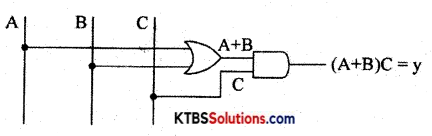(b) Answer any four questions. (4 × 5 = 20)

Question 32.
Explain the following terms of an A.C signal
(1) Instantaneous Voltage
(2) Peak or Maximum Voltage
(3) Peak to Peak Voltage
(4) RMS Value
(5) Time Period of the signal
Definition of

1. The frequency of AC is the number of AC cycles completed in one second.
2. The time period of AC is the time taken to complete one cycle.
3. The peak value of AC is the maximum voltage or current of the ac in its one of half cycles.
4. RMS value of ac is the equivalent steady DC value which gives the same heating effect as the same rate in a given resistor.
5. The peak to the peak value of AC is the maximum value attained in both positive and negative half cycles of AC.

Question 33.
(a) Explain the construction of the carbon film resistor. (4M)
(b) On an SMD resistor component it is printed as 4R7. What is its resistance value? (1M)
(a) Construction of carbon film resistor.
(b)R = 4.7Ω.Question 34.
(a) With a labeled diagram explain the working of a moving coil loudspeaker. (3M)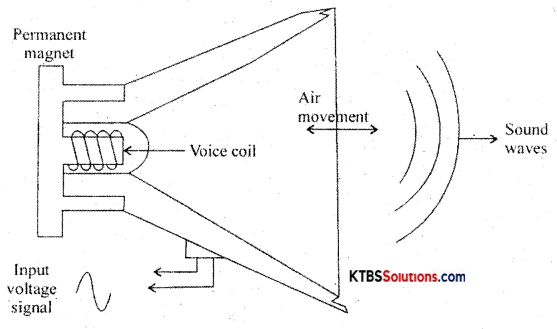(b) What are NTC and PTC? (2M)

• NTC- Negative Temperature Coefficient of Resistance.
• PTC- Positive Temperature Co-efficient of Resistance.

Question 35.
(a) For an RLC A.C circuit derive an expression for impedance. (3M)
(b) What is the condition for Resonance? Write the expression for resonant frequency. (2M)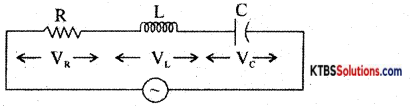Derivation for Z : Z = $$\sqrt{R^{2}+\left(X_{L}-X_{C}\right)^{2}}$$
(b)XL = Xc
f = $$\frac{1}{2 \pi \sqrt{\mathrm{LC}}}$$

Question 36.
Explain Demorgan’s theorems with truth tables.